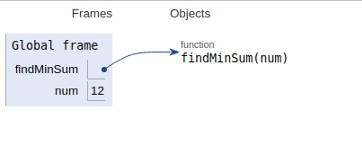# Python Program for Find minimum sum of factors of number

## Problem statement

Given a number input , find the minimum sum of factors of the given number.

Here we will compute all the factors and their corresponding sum and then find the minimum among them.

So to find the minimum sum of the product of number, we find the sum of prime factors of the product.

Here is the iterative implementation for the problem −

## Example

Live Demo

#iterative approach
def findMinSum(num):
sum_ = 0
# Find factors of number and add to the sum
i = 2
while(i * i <= num):
while(num % i == 0):
sum_ += i
num /= i
i += 1
sum_ += num
return sum_
# Driver Code
num = 12
print (findMinSum(num))

## Output

7

All the variables are declared in the global frame as shown in the figure given below −## Conclusion

In this article, we learned about the approach to Find the minimum sum of factors of a number.